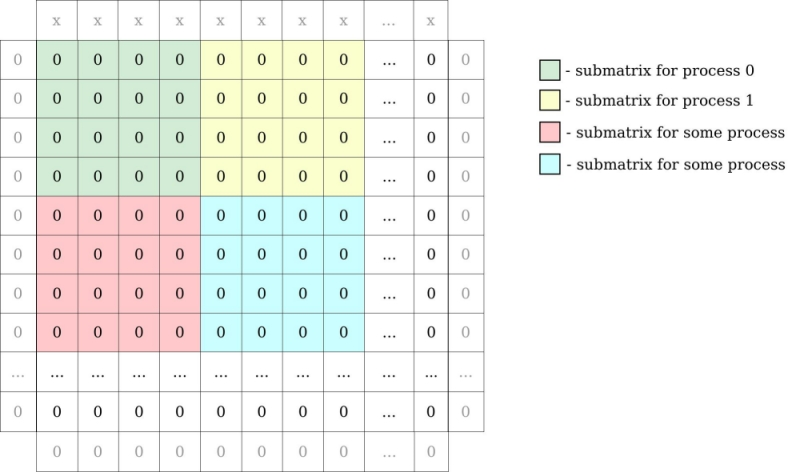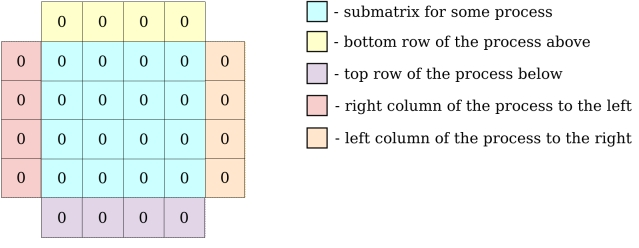# PDI 1.0.1

## Data exchange made easy

PDI example

PDI example application can be found in repository in the `example` directory. The source code is written in C, Fortran and Python.

The example has a single source file (for each supported programming language), but specification tree to each plugin. Just by replacing the configuration file, the example program will use different libraries and different I/O operations.

The example implements simple Heat equation algorithm in 2 dimensional space.

# Heat algorithm

Each element in a matrix is a point with temperature. In each iteration every cell is calculated by an weighted average of itself and neighbour cells (top, bottom, left and right):

matrix[i][j] = 0.500 * matrix[i][j] +
0.125 * matrix[i-1][j] +
0.125 * matrix[i+1][j] +
0.125 * matrix[i][j-1] +
0.125 * matrix[i][j+1];

To not override the cells while processing we need to create temporary matrix to save results and then move result to original matrix (`cur` and `next` in source file). Above calculations are written in `iter` function.

Now we can add a MPI to our algorithm. Let's split that matrix by MPI processes. Each process will compute part of global matrix.

For example: matrix 16 x 16 integers and 16 MPI processes gives submatrices of 4 x 4 integers for every process. We have to add to our global matrix one row above and below, column to the left and right to be able to compute border cells. In our example row on the top has some value (”x”) bigger than 0 (representing source of heat):MPI processes need to exchange information about their local matrix border cells (communicate with neighbours to exchange row/column of matrix). Each MPI process will have a local matrix:All the communications instructions are written in `exchange` function.

# PDI integration

Now, when we know the algorithm, we can focus on analysing `decl_hdf5.yaml` specification tree. Fisrt 3 maps defined will not be seen to PDI:

duration: 0.75
datasize: [60, 12]
parallelism: { height: 3, width: 1 }
• `duration` is the value in seconds how long the application will run.
• `datasize` is size of our global matrix.
• `parallelism` defines the number of MPI processes in each dimension.

Next, we have defined `data` and `metadata`:

pdi:
iter: int
dsize: { size: 2, type: array, subtype: int }
psize: { size: 2, type: array, subtype: int }
pcoord: { size: 2, type: array, subtype: int }
data:
main_field: { size: [ '\$dsize', '\$dsize' ], type: array, subtype: double }

In source file we will extract the `pdi` map and pass it as PDI_init argument.

• `iter` will hold the current iteration number.
• `dsize` will hold the size of local matrix of each MPI process.
• `psize` will hold number of processes in dimensions.
• `pcoord` will hold coordinates for each process.
• `main_filed` is the local matrix for each process.

Let's take a closer look at C source code.

PDI_init(PC_get(conf, ".pdi"));

As mentioned before, we extract the `pdi` subtree and pass it to PDI_init.

We did not defined `mpi_comm` data in yaml, so this line will have no effect:

PDI_expose("mpi_comm", &main_comm, PDI_INOUT);

The same goes for all PDI calls with data we didn't defined.

int dsize;
PC_int(PC_get(conf, ".datasize"), &longval); dsize = longval;
PC_int(PC_get(conf, ".datasize"), &longval); dsize = longval;

Here we are reading global matrix size from specification tree. Similar with parallelism and duration.

After calculating the local matrix sizes and coordinates, we expose them:

PDI_expose("dsize", dsize, PDI_OUT);
PDI_expose("psize", psize, PDI_OUT);
PDI_expose("pcoord", pcoord, PDI_OUT);

At the beginning of each iteration, we call multiexpose:

PDI_multi_expose("newiter",
"iter", &ii, PDI_INOUT,
"main_field", cur, PDI_INOUT,
NULL);

Above instruction will share `iter` and `main_field`, call `newiter` event and then reclaim `main_field` and `iter`. This is the place when plugins will read/write our data.

We have covered the logic behind the PDI example. Now you can start the Hands-on tutorial.